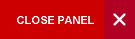## ECON3032: STATISTICAL ESTIMATION AND INFERENCE FOR BUSINESS AND SOCIAL SCIENCES

Outline: Concepts of estimation, estimate and estimator - point estimate and interval estimation - desirable properties of a point estimator; estimation theory (mathematical definition/ concept of desirable properties of the estimators (techniques of estimation of parameters, method of moments, method of maximum likelihood and Minimum Variance Unbiased (MVU) estimators Cramer-Rao inequality etc., confidence intervals for the mean, variance and differences between means etc. - applications; hypothesis testing (basic concepts in hypothesis testing type I and type II errors, level of significance etc., Neyman-Pearson lemma - Power function of a test, likelihood ratio tests - Application in terms of normal, t, F and Chi-square tests etc. - Sequential analysis; regression and correlation (estimation in linear, bivariate and multiple regression - Least squares estimation - method of estimation of correlation coefficients.

Pre-requisites: ECON3031 or MATH2404 (Anti-requisite: STAT2001)

Semester: 2

Lecturer: Mr Rayon Cameron

Office Hours: N/A

Office Location: